Learning Objective

In this lesson we will learn about the different types of acid-base reactions and the products they form.

Learning Outcomes

By the end of this lesson you will be able to:

• Identify acid-base reactions.
• Write the generalised chemical equations for different types of acid-base reactions.
• Determine the products of acid-base reactions.

Acid-Base Reactions

1 | Introduction to Acid-Base Reactions

2 | Acids in Solution

3 | Bases in Solution

4 | Neutralisation Reactions

5 | Determining the Type of Salt Produced in Acid-Base Reactions

6 | Reactions Between Acids and Metal Hydroxides

7 | Reactions Between Acids and Metal Oxides

8 | Reactions Between Acids and Metal Carbonates

9 | Reactions Between Acids and Metal Hydrogen Carbonates

10 | Summary

Click images to preview the worksheet for this lesson and the Year 9 Chemistry Workbook (PDF and print versions)

## Introduction to Acid-Base Reactions

• There are four main types of acid-base reactions, depending on the type of base and the products formed:
• Reactions between acids and metal hydroxides.
• Reactions between acids and metal oxides.
• Reactions between acids and metal carbonates.
• Reactions between acids and metal hydrogen carbonates.
• Reaction types 1 and 2 produce a salt and water.
• Reaction types 3 and 4 produce a salt, water and carbon dioxide.
• Acid-base reactions are also known as neutralisation reactions.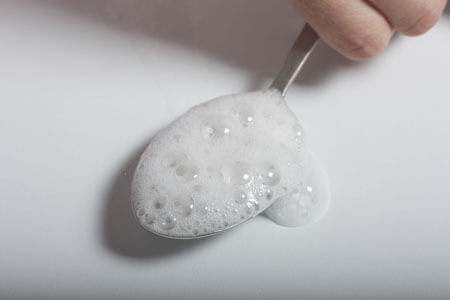Mixing vinegar (acetic acid) and baking soda (sodium hydrogen carbonate) is an acid-base reaction.

## Acids in Solution

• Acids are substances that produce hydrogen ions (H+) in solution.
• The formation of acid solutions can be shown by the following generalised formula:• Examples
• ▸ Hydrochloric acid:  HCl    H+  +  Cl
• ▸ Nitric acid:  HNO3    H+  +  NO3
• ▸ Acetic acid:  CH3COOH    H+  +  CH3COO

## Bases in Solution

• Bases are substances that produce hydroxide ions (OH) in solution.
• They are ionic compounds, where the negative ion is one of the following:
• ▸ Hydroxide (OH)
• ▸ Oxide (O2–)
• ▸ Carbonate (CO32–)
• ▸ Hydrogen carbonate (HCO3)
• The formation of base solutions from hydroxides can be shown by the following generalised formula: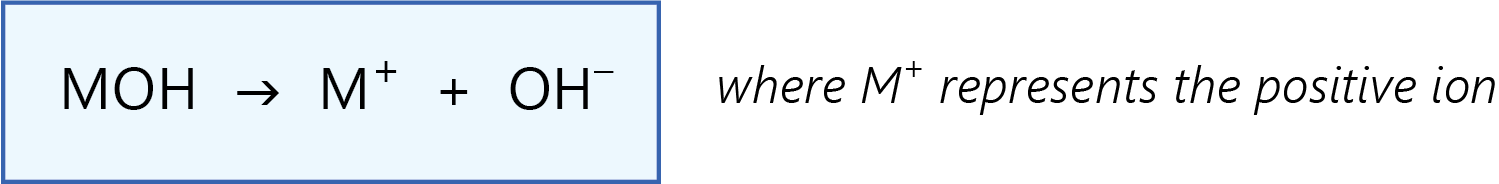• Example
• ▸ Sodium hydroxide:  NaOH    Na+  +  OH
• The formation of base solutions from oxides, carbonates and hydrogen carbonates is more complex, but result from reactions between these substances and water.
• Examples
• ▸ Sodium oxide:  Na2O  +  H2O    Na+  +  OH
• ▸ Sodium carbonate:  Na2CO3  +  H2O    H2CO3  +  Na+  +  OH
• ▸ Sodium hydrogen carbonate:  NaHCO3  +  H2O    H2CO3  +  Na+  +  OH

## Neutralisation Reactions

• Chemical reactions between acids and bases are known as neutralisation reactions.
• This is because, if they are mixed in equivalent proportions, there will be an equal amount of hydrogen ions and hydroxide ions, and therefore the resulting solution will be neutral. (Sometimes the products of the reaction react with the water, resulting in a solution that is acidic or basic).
• Neutralisation reactions result in the formation of ionic compounds known as salts.
• Examples of salts include sodium chloride (NaCl), magnesium sulfate (MgSO4) and potassium acetate (KCH3COO).
• Depending on the type of base, neutralisation reactions will also produce water, or both water and carbon dioxide.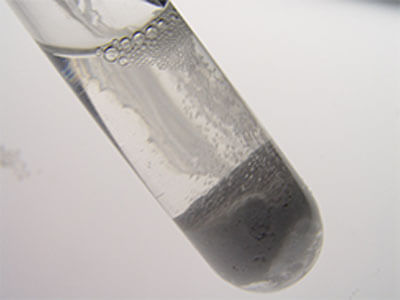The reaction between nitric acid and strontium carbonate as an example of a neutralisation reaction.

(Image: LoyalSoldier, Wikimedia Commons)

## Determining the Type of Salt Produced in Acid-Base Reactions

• The type of salt produced in acid-base reactions depends on both the acid and the base.

### The Positive Ion

• The positive ion of the salt produced in acid-base reactions is determined by the positive ion of the base.
• Examples
• Magnesium hydroxide, magnesium oxide, magnesium carbonate and magnesium hydrogen carbonate will all form magnesium salts when reacting with acids.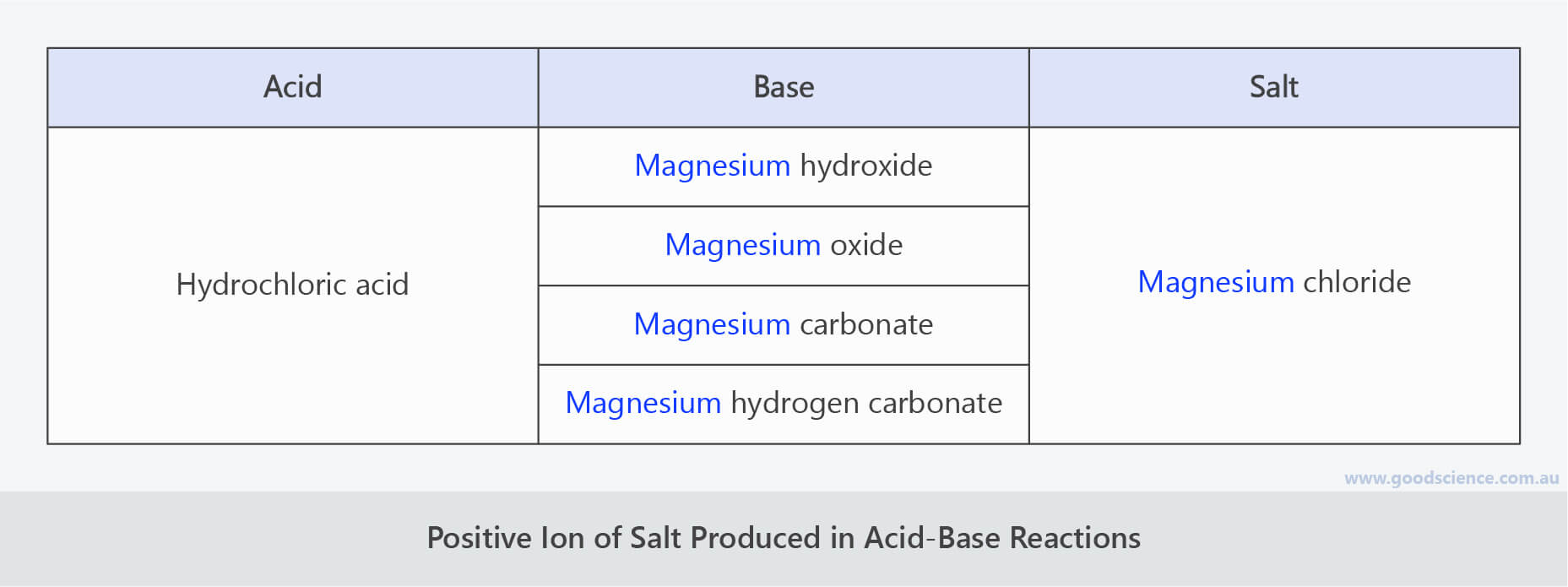The positive ion of the salt produced in an acid-base reaction is determined by the base.

### The Negative Ion

• The negative ion of the salt produced in acid-base reactions is determined by the negative ion of the acid.
• Examples
• ▸ Hydrochloric acid forms chloride salts.
• ▸ Sulfuric acid forms sulfate salts.
• ▸ Nitric acid forms nitrate salts.
• ▸ Acetic acid forms acetate salts.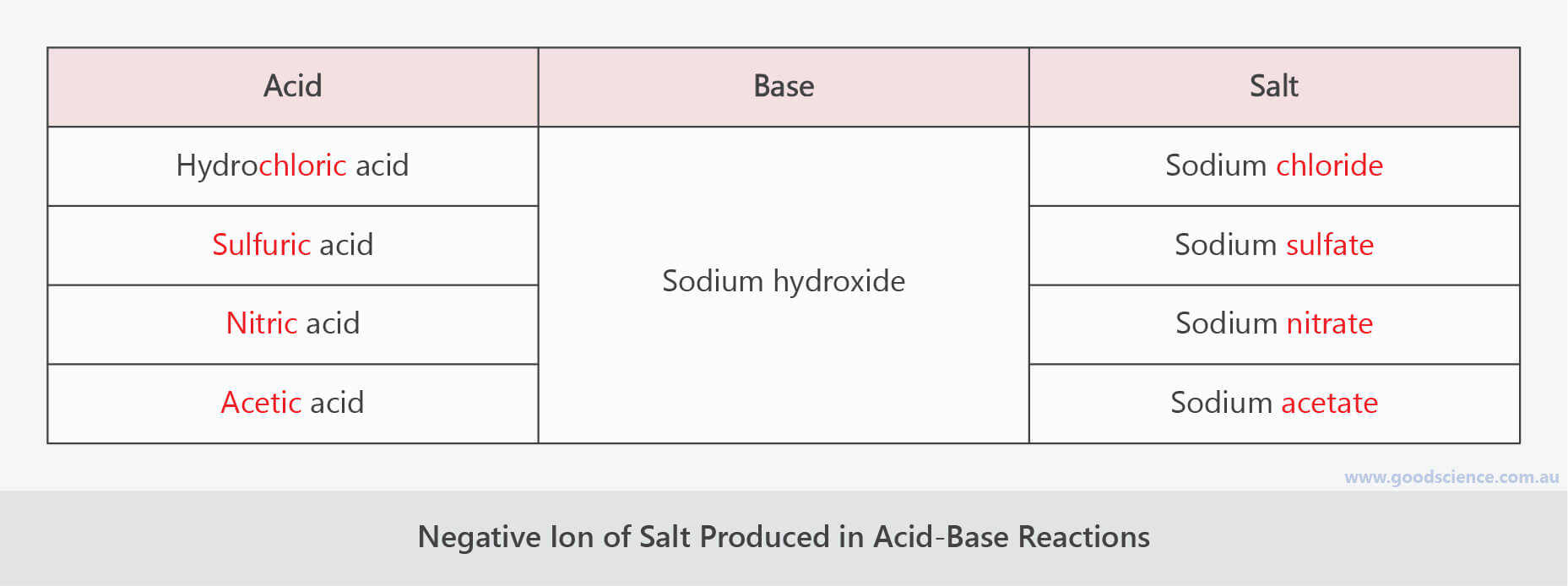The negative ion of the salt produced in an acid-base reaction is determined by the acid.

### Ammonium Salts

• The ammonium ion (NH4+) is a bit unique. It is a polyatomic ion with a positive charge.
• Ammonium can form the positive ion in bases and salts.
• Therefore, the four types of acid-base reactions described below could have ammonia as the positive ion, rather than a metal.
• Example
• ▸ HCl  +  NH4OH    NH4Cl  +  H2O

## Reactions Between Acids and Metal Hydroxides

• Acids react with metal hydroxides to produce a salt and water.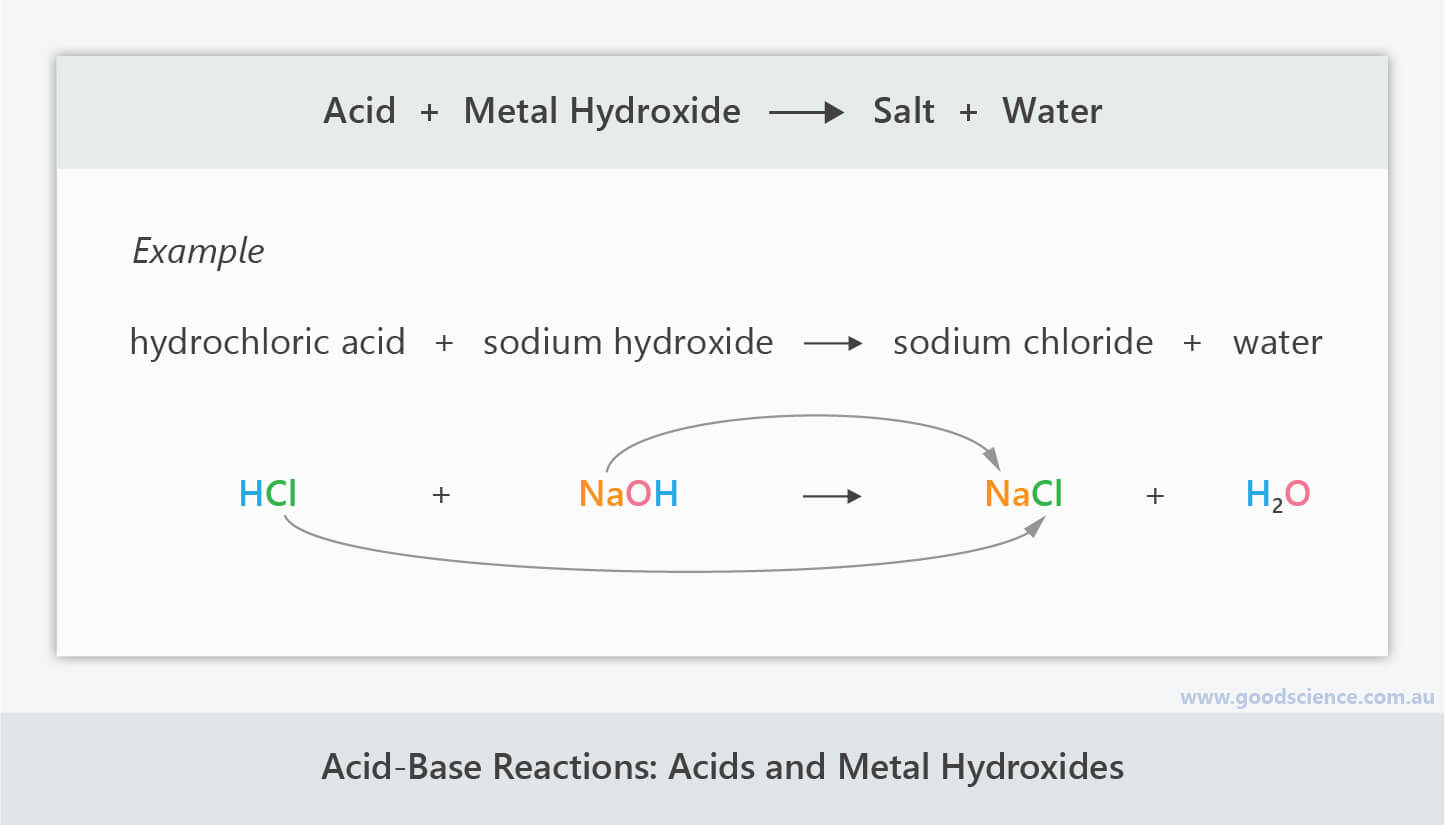Acids and metal hydroxides react to form a salt and water.

## Reactions Between Acids and Metal Oxides

• Acids react with metal oxides to produce a salt and water.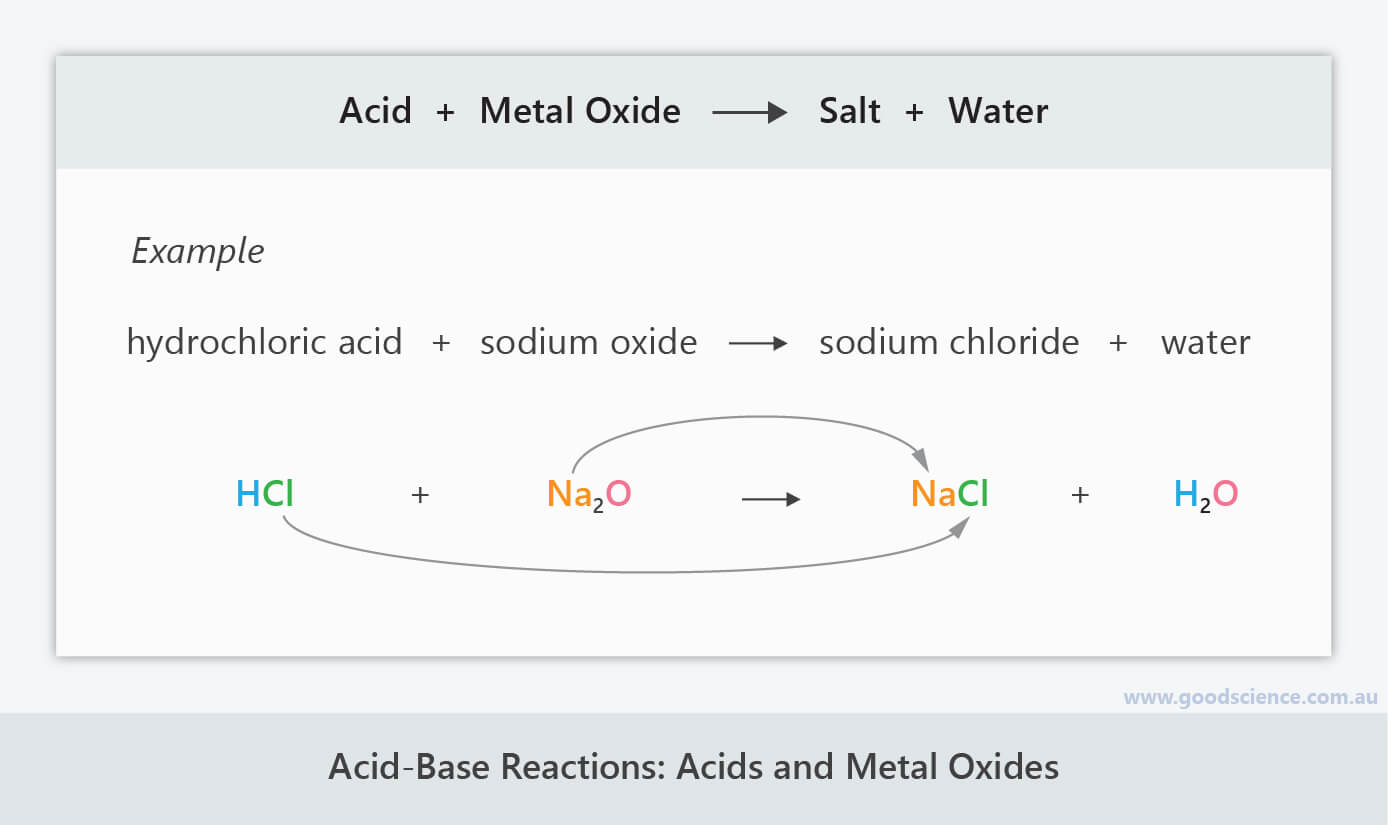Acids and metal oxides react to form a salt and water.

## Reactions Between Acids and Metal Carbonates

• Acids react with metal carbonates to produce a salt, water and carbon dioxide.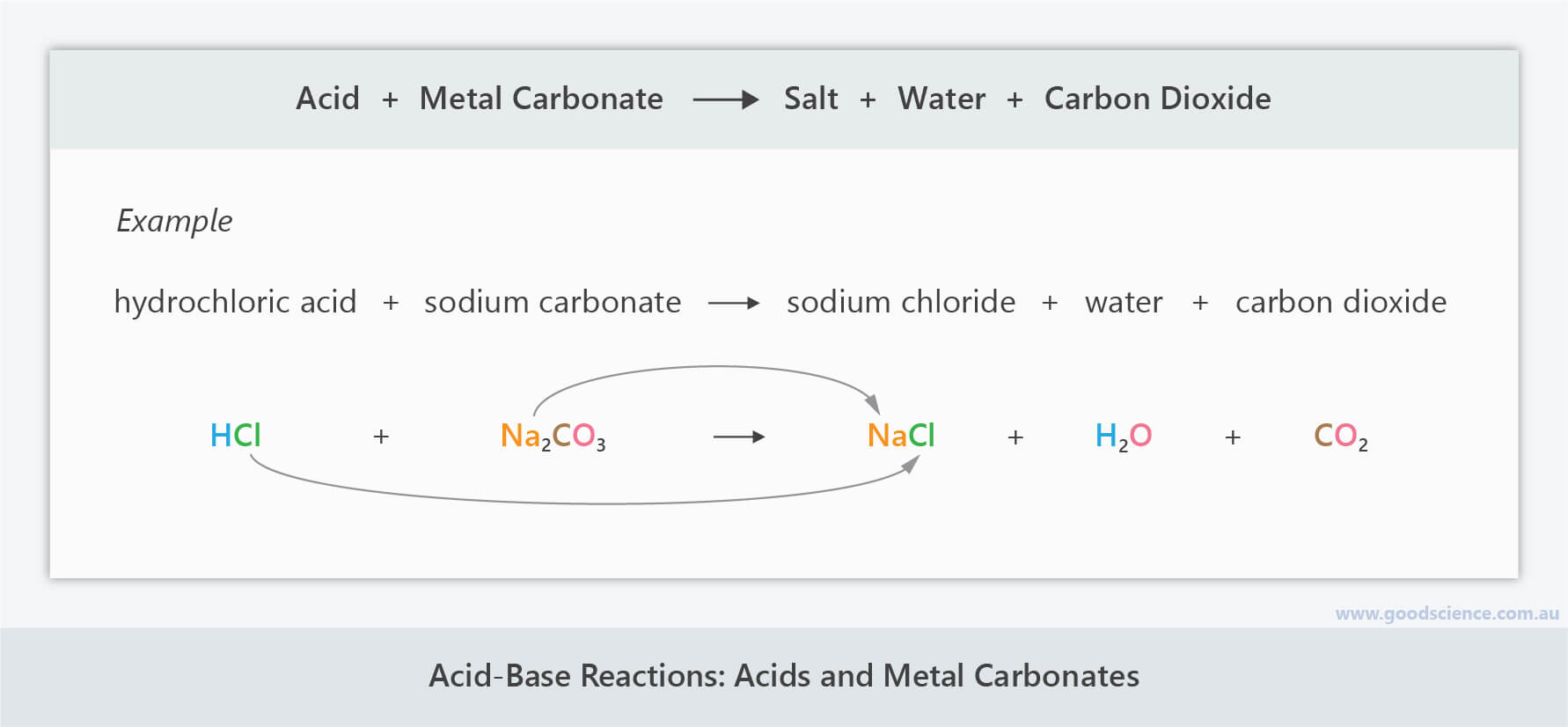Acids and metal carbonates react to form a salt, water and carbon dioxide.

## Reactions Between Acids and Metal Hydrogen Carbonates

• Acids react with metal hydrogen carbonates to produce a salt, water and carbon dioxide.Acids and metal hydrogen carbonates react to form a salt, water and carbon dioxide.

## Summary

• Chemical reactions between acids and bases are known as neutralisation reactions.
• Neutralisation reactions result in the formation of ionic compounds known as salts.
• Depending on the type of base, neutralisation reactions will also produce water, or both water and carbon dioxide.
• There are four main types of acid-base reactions, depending on the type of base and the products formed.
• Reactions between acids and metal hydroxides:
• Acid  +  Metal Hydroxide    Salt  +  Water
• Reactions between acids and metal oxides:
• Acid  +  Metal Oxide    Salt  +  Water
• Reactions between acids and metal carbonates:
• Acid  +  Metal Carbonate    Salt  +  Water  +  Carbon Dioxide
• Reactions between acids and metal hydrogen carbonates:
• Acid  +  Metal Hydrogen Carbonate    Salt  +  Water  +  Carbon Dioxide(Image: katerha, Wikimedia Commons)

(Header image: Alessandro e Damiano, Wikimedia Commons)

Click images to preview the worksheet for this lesson and the Year 9 Chemistry Workbook (PDF and print versions)